# Numerical Investigation for Single-Phase and Two-Phase Flow in Duct Banks with Multi Types of Vortex Generators

Title: Numerical Investigation for Single-Phase and Two-Phase Flow in Duct Banks with Multi Types of Vortex Generators

Corresponding email:

Ibrahim, A.Q., Alturaihi, R.S., 2023. Numerical Investigation for Single-Phase and Two-Phase Flow in Duct Banks with Multi Types of Vortex Generators. International Journal of Technology. Volume 14(3), pp. 484-500

244
 Adnan Qahtan Ibrahim 1. Department of Mechanical Engineering, Faculty of Engineering, University of Babylon, Hilla, Babylon, 51002, Iraq, 2. Automotive Department, College of Engineering/AL-Musaib, University of Babylon, Riyadh Sabah Alturaihi 1. Department of Mechanical Engineering, Faculty of Engineering, University of Babylon, Hilla, Babylon, 51002, Iraq
Email to Corresponding Author

Abstract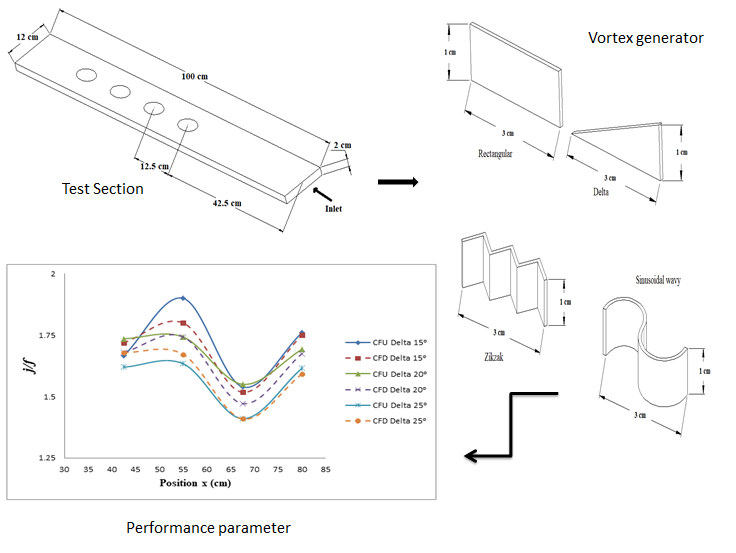This paper examines the numerical work of the thermal convection over tube banks with winglets through the heat exchanger in two parts under turbulent conditions. The first section investigates the influence of two-phase (water and air) flow on the performance of two kinds of vortex generators (Delta and Rectangular) winglets across the oval tube banks. The second section studied the performance of four types of winglets (Delta, Rectangular, Zikzak, and Sinusoidal wavy), circular and oval tubes, forward and downward configurations, and various angles of attack (15°, 20°, and 25°) in two-phase flow. Delta winglets provide the highest performance at an attack angle of 15° for oval tube banks in a two-phase flow with a moderate turbulent flow rate.

Duct Banks; Heat exchanger; Sinusoidal winglets; Zikzak winglets

Introduction

In numerous industrial processes, heat convection is essential in heating and cooling operations. At high flow velocities, a two-phase flow is utilized to minimize pressure losses in the duct. Over the last two decades, researchers have focused on winglets' ability to generate interconnected vortices or swirling flow parallel to the flow orientation. These winglets have been studied to enhance the Heat convection effectiveness of various heat exchangers via air- or gas-side heat transfer. (Fiebig, 1998; Fiebig, Valencia, and Mitra, 1993) explained flow with winglets could lead to a massive increase in heat convection coefficient in the laminar duct flows, increasing transverse vortex generators (VGs). It was found that an inline tube configuration enhanced the heat convection rate by 55–65 %, with a comparable pressure drop of 20–45 %. Lau, Meiritz, and Ram (1999) published the results of an experimental work investigating the movement in a turbulent duct flow with heat and momentum using Vortex generators. Oval tubes have several advantages over circular tubes in lowering pressure drop and reducing the wake zone. As a result, when vortex generators are combined with oval tubes, it is possible to get increased thermal performance without considerably raising the pressure drop (He and Zhang, 2012). Compared to the Baseline instance, the vortex generator exhibits a promising improvement in heat convection coefficients and a significant penalty in pressure loss (Sahel, Benzeguir, and Baki, 2015). The heat convection coefficient in the region of the wake behind the tube can be increased by up to 240% in the addition of winglet-kind longitudinal VGs. The overall channel heat convection coefficient has significantly increased, according to the existing findings. The improvement has a lot of potential for reducing the heat exchanger size (Lu and Zhou, 2016; Tiwari et al., 2003; Biswas, Mitra, and Fiebig, 1994). The overall heat transfer coefficient for both laminar and turbulent flows increases as the number of pairs of VGs increased, based on the number of rows difference (Syaiful et al., 2017). The geometry of the tube banks impacts the thermo-hydraulic characteristics of a fin and tube heat exchanger (FTHE) directly (Sahel, Ameur, and Mellal, 2020) and a reduction in the heat convection coefficient as the flat tube's size increases (Sahel, Ameur, and Boudaoud, 2019). A study by Susanto et al. (2020) showed that the heat transfer rate improves more smoothly; where the influence of convection walls is minimal, the local Nusselt number (Nu) grows and the velocity profile increased marginally. The addition of flat vortex generators increases the heat convection coefficient by approximately 62.53% (Sahel, Ameur, and Alem, 2021). Augmentation of heat convection occurs with curved rectangular winglet VGs for flow in a rectangular channel (Naik, Tiwari, and Kim, 2022) and for flow across three rows of cylindrical tubes installed on a flat plate with height-averaged (Nu) and perimeter-averaged (Nu) variances along the height of a cylinder. (Naik and Tiwari, 2017; Naik and Tiwari, 2018). With upstream rectangular winglet pair positions, performance is improved (Naik and Tiwari, 2020a). In recent years, researchers have investigated several additional areas for vortex generator forced convection enhancement, including the addition of bulges, dimples, and punched delta winglets as vortex generators are all geometric features. The collected data indicate that a tube incidence angle of 20° is the optimal structure for eliminating the hot areas among the tubes and increasing the heat convection coefficient by 13% according to the base scenario (Abdoune et al., 2021). It is known that tube diameter and height variances impact the convection heat coefficient value (Susmiati et al., 2022). Inserts of various models to Vortex generators (Oneissi et al., 2018; Tang et al., 2016; Boonloi and Jedsadaratanachai, 2016), the use of combined winglets (Tamna et al., 2016), delta winglets with Nanofluids (Ahmed et al., 2017), built-in interrupted delta-winglet (Wu et al., 2016), the application of VGs to various kinds of heat exchangers (Garelli et al., 2019; Song and Tagawa, 2018), and enhancement of heat convection have been documented for angles of attack ranging from 0° to 45o. In contrast, the heat convection coefficient decreases for angles of attack ranging from 135° to 180° (Afshari, Zavaragh, and Nicola, 2019). In this study, the air is used as a second phase with water to increase vortex generators' efficiency by reducing pressure loss in the duct.

Experimental Methods

The Geometric Model

2.1. Modelling of Flow and Boundary Condition

The characteristics of the problem and boundary condition for the single and two phase-flows can be shown in Figure 1. The rectangular duct inlet is represented as the fluid inlet's superficial velocities. The oval tube wall is exposed to constant heat flux, while the outlet pressure is represented at the outlet of the rectangular duct. The remaining portion of the duct walls is put to be adiabatic.

The heat convection coefficient and the temperature distribution were investigated in the duct by using various values for the discharges of water and air with different shapes and positions of the vortex generators. ANSYS-Fluent 19.0 is used to evaluate the flow characteristics of water flow and mixture (water-air) flow through the banks of the tubes with vortex generators. Fluent shows temperature distribution, pressure gradient, and velocity for two-phase and single-phase flow through the tube banks.

Fluent is a fluid flow simulation software suite that solves fluid flow problems using computational fluid dynamics (CFD). It solves a fluid's governing equations by using the finite volume method. Fluent's fluid problem scheme is defined by momentum, mass, and energy conservation laws. This law defines a finite volume-based discretization of a partial differential equation. These parameters pass in the course of the rectangular duct for fluid flow over oval tube banks with and without winglets. Computational fluid dynamics (CFD) is the branch of fluid dynamics that investigates the issue by calculating and giving practical methods for reproducing natural flow by solving the governing equations numerically (Abdulnaser, 2009).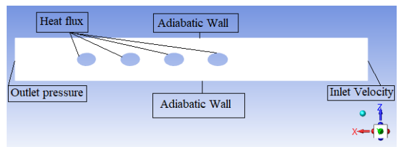Figure 1 Boundary Condition

The boundary conditions of the single-phase and two-phase flow systems are presented in Table 1.

Table 1 Boundary conditions

 Zone Fluid Energy Inlet Velocity 293 k Tube walls No-slip 21883.8 w/m2 Duct walls Symmetry Symmetry Winglets No-slip Adiabatic Outlet Pressure Adiabatic

2.2. The Geometry of the Testing Section

In order to simulate the system, it has been modeled as a 3-D model using Solid Works 2018 combined with Ansys Workbench 19.0. The model has been drawn as a rectangular shape, and its dimensions are (12 cm × 2 cm × 100 cm). The geometry of the testing section is set to be fluid, as shown in Figure 2. Using the diameter of the tube (D) as the characteristic length scale, all dimensions of the duct are calculated as L = 10D, W = 1.2D, and H = 0.2 D, respectively.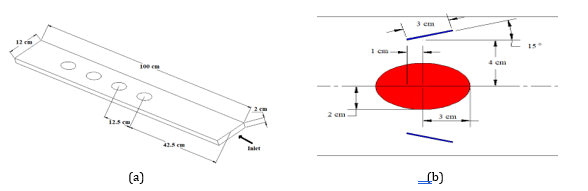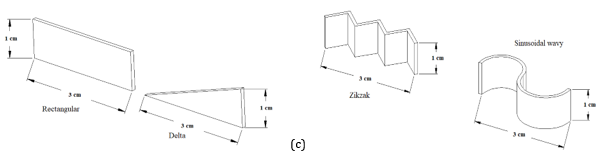Figure 2 (a) Computational domain for test section; (b) Vortex generator placement with oval tube; (c) Four types of vortex generators

2.3. Mesh Generation

Because there are so many mesh types to choose from, it is essential to consider factors like flow field, geometry, and complexity when choosing which mesh to use. The required CPU time, solution accuracy, and convergence rate are all influenced by the size and kind of mesh (Bakker, 2006). In this work, the meshing procedure is performed in the Ansys Workbench 19.0 application using Quadrilateral structured grid elements. The meshing sizes for maximum and minimum meshing sizes are set to be equal (0.001 m) for oval and circular tubes, as shown in Figure 3. Table 2 shows how many elements and nodes each situation in this study contains for oval and circular tubes, respectively.

Table 2 The number of elements and nodes

 Case Nodes No. Elements No. Without Vortex 202860 181440 Delta 124767 624620 Rectangular 124831 624663 Zikzak 124826 624657 Sinusoidal 134436 674249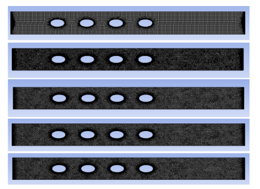Figure 3 The Mesh of oval tubes

2.4. Grid independence

A grid-independent solution is required to enhance the precision of the computations. The present work on grid independence consists of three parts: convergence index, grid refinement, and General Richardson. Two various winglet positions relative to the center of each tube are used. For forward (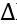X = -1,Y = ±4), downward (X = 1,Y = ±4) of each tube with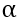= 15° and Re = 3643.5.

3.    Governing Equations

The challenge entails solving the flow field and heat convection problems associated with a bank of oval and circular tubes fitted with winglets in a rectangle duct under a transient state. Vortex generators are added to the duct to improve heat transfer. A computational examination is necessary because of how their addiction impacts the flow field and heat convection. The flow field must be resolved to establish the ideal heater diameter and form for the vortex generators.

3.1. Continuity Equation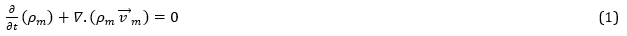3.2. Momentum Equation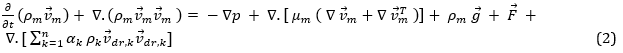3.3. Energy Equation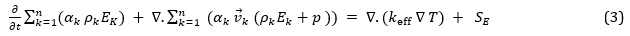4.    Turbulence Model

Models are utilized to have the capacity for characterizing and predicting the physics of the multiphase flow. Also, these models are suitable for different applications that have multiphase flow. Some demonstrating approaches are the Euler-Lagrange approach, the Volume of fluid approach, the Euler-Euler approach, and dispersed phase modeling. The Euler-Lagrange method is computationally expensive and is appropriate for flows with a small volume percentage of the dispersed phase. The (k-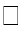) standard model is utilized in place of the (k-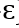) model in this work due to the (k-) model's poor prediction of rotating and swirling flows, as well as fully developed flows in the rectangular ducts (Shbeeb and Mahdi, 2016).

Also, the (k-) standard turbulence model will be used to simulate the flow-through test section. The single and two-phase flows are modeled by combining the model with various parameters based on the testing factors and the outcomes of the experiments to compare and validate the CFD results (Vejahati et al., 2009; Fluent, 2006).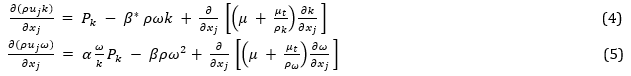5.    Performance parameterwhere j is the Colburn factor, and f is the coefficient of friction (Chu, He, and Tao, 2009).

6.    Model validation

The computational fluid dynamics model validation by numerical simulations of flow through the heat exchanger with an intake of the Reynolds number between 600 and 3000 were compared to the numerical outcomes of (Fiebig, Valencia, and Mitra, 1993).

The maximum errors between the present model findings and the numerical outcomes of (Fiebig, Valencia, and Mitra, 1993) are 4.35% for Nu and 5.825% for f. The model validation results are shown in Figure 4. From the above analyses, the high degree of agreement between these outcomes illustrates the model's reliability in precisely forecasting the flow structure and heat convection properties.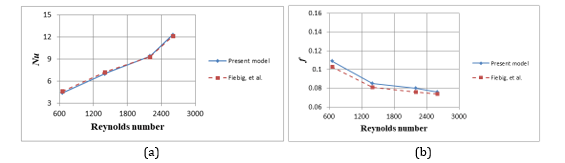Figure 4 Validation of the present model with the germane work (Fiebig, Valencia, and Mitra, 1993) for various values of Re (a) Nu and (b)

Results and Discussion

Figures 5 to 19 show the numerical results of the increased water-air flow rates on the temperature gradient at various locations in the duct with a constant electrical power of (110 W) as a heat flux. In this study, the performance of VGs was investigated based on the temperature gradient between the fluid flow and the surface of the tube bank, which corresponded to the pressure loss inside the duct. The best performance at the lowest temperature difference and the lowest pressure drops, with other parameters remaining kept constant. The Nusselt number (Nu) was directly proportional to the coefficient of heat convection. When the temperature gradient between single or two-phase flow and the surfaces of tube banks increased, the heat convection coefficient decreased, and vice versa. Also, the friction coefficients (f) were directly proportional to the pressure drop in the duct, according to Equation 7.

7.1. Effect of the phase

7.1.1.   Single-phase flow

Figure 5 illustrates the temperature gradient between water flow and oval tube surfaces at various locations in the duct without winglets, with Delta winglets, and with Rectangular winglets for three different water flow rates (15, 17.5, and 20 L/min). Observed from this figure that the temperature gradient decreased as the water flow rate increased. These results agree with Chu, He, and Tao (2009), and Haque and Rahman (2020) for reduced temperature gradient at the water flow velocity increased; therefore, the heat convection coefficient increased. When the water flow rate increases, the flow velocity increases, ultimately enhancing the heat convection coefficient.

Figure 6 illustrates the entrance and exit pressure for water flow at many points in the duct without winglets, with Delta winglets, and with Rectangular winglets for three different water flow rates (15, 17.5, and 20 L/min). The pressure loss in the duct raised as the discharge of water increased, and these results agree with Chu, He, and Tao (2009). When the water flow rate increases, the pressure drop decreases, which reduces the coefficients of friction.

Adding vortex generators to the duct can augment the heat convection coefficient by raising the velocity of single-phase flow and the turbulence intensity within the duct. As the flow velocity increases, the temperature gradient reduces. When flow is oriented toward the oval tube's surface, the temperature gradient is inversely proportional to flow velocity.

Figure 7a illustrates the fluctuation of a temperature gradient with Reynolds number in water flow across the bank of oval tubes without winglets generators. Owing to a decrease in the temperature gradient between the water flow and surfaces of the oval tube, the heat convection coefficient improved as the Re number raised.

Figure (7b) represents the fluctuation of the pressure reduction with Reynolds number in water flow over oval tube banks without winglets. As the Reynolds number grew, the duct pressure losses were reduced.

Figure (7c) represents the Reynolds number performance parameter for water flow over oval tube banks without winglets. As the Re number climbed, the performance parameter improved because the pressure in the duct and the temperature gradient between the water flow and the surfaces of the oval tube decreased. The delta winglets' performance is greater than that of the other two vortex generators. These results concur with the reports of Haque and Rahman (2020), and Naik and Tiwari (2020b) which examined the influence of different vortex generator forms on heat flow in the duct and showed that Delta winglets provide the highest performance.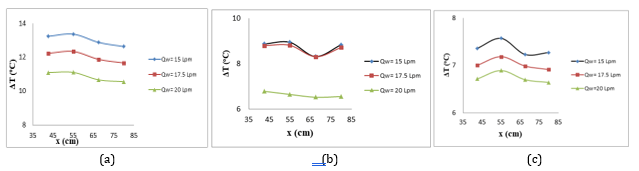Figure 5 The temperature gradient between water flow and surfaces of an oval tube for various water flow rates (15, 17.5, and 20 L/min) for single-phase flow (a) without winglets (b) with Delta winglets (c) with Rectangular winglets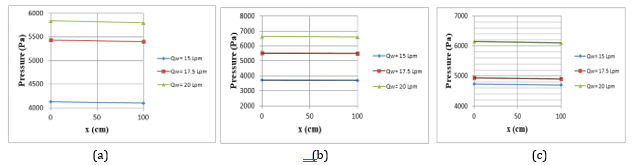Figure 6 Inlet and Outlet pressure in the duct with an oval tube for single-phase flow (a) without winglets (b) with Delta winglets (c) with Rectangular winglets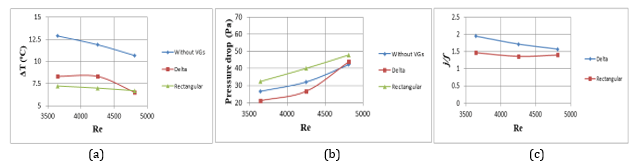Figure 7 (a) The temperature gradient with Delta and Rectangular winglets for single-phase flow over oval tube (b) Pressure drop (c) Performance parameter

7.1.2.   Two-phase flow

Figure 8 indicates that at a constant discharge of water, the temperature gradient between mixture (water-air) flow and surfaces of oval tubes increased. At these points, the airflow rate increases (8.33, 16.67, and 25 L/min) with a constant water flow and heat flux. When the flow rate of water and air flow is increased without winglets, the heat convection rate drops, and the temperature gradient becomes less significant. The heat convection coefficient improved as the temperature difference reduced because the winglets concentrated the working fluid on the tube surface with Delta winglets and Rectangular winglets used in the duct.

Figure 9 represents the inlet and outlet pressure in the duct for water-air flow at various points. At these points, the airflow rate increases (8.33, 16.67, and 25 L/min) with a constant flow rate of water and heat flux. The pressure losses without winglets in the duct are reduced. Increased pressure drops compared to Delta winglets, and Rectangular winglets are used in the duct that is not utilized. In addition, the pressure drops in the duct are minimized due to growth in the flow rate of water-air Delta winglets. This is in accordance with the findings of Chu, He, and Tao (2009) and Haque and Rahman (2020).

In addition, the pressure drop is reduced when the discharge of mixture (water-air) flow is increased in the absence of winglets. When rectangular VGs are used in the duct, the pressure losses are more significant than not. In addition, when the flow rate of water-air increases with rectangular winglets, the pressure losses in the duct are decreased. There is good agreement with that of Haque and Rahman (2020) and Naik and Tiwari (2020b).

Adding winglets to the duct can raise the surface velocity of water-air flow, raising the heat convection coefficient by generating intense turbulence. The temperature gradient decreases as the flow velocity increases. When the flow is directed toward the surfaces of the oval tube, the temperature gradient is inversely related to the flow velocity. The airflow rate increased from (8.33, 16.67, and 25 L/min) with a constant flow rate of water and heat power of (110 W).

The effect of the Reynolds number for water-air flow over oval tube banks in a turbulent region on heat convection rate and pressure reduction is essential for optimal design and location of vortex formation. Due to the decrease in pressure and temperature gradient between water-air flow and surfaces of the oval tube in the duct, the performance parameter was improved when the Re number of water was lowered. The Re number of air grew as the air flow rate raised (8.33, 16.67, and 25 L/min), but the water flow rate remained constant.

The variance of the temperature gradient with Re number in water-air flow over oval tube banks without winglets, with Delta winglets, and with Rectangular winglets are represented in Figure 10. At a constant flow rate of water, the heat convection coefficient increases as the temperature gradient between the water-air flow and surfaces of the oval tube decreases.

The variance of the drop in pressure with the Re number in water-air flow over oval tube banks without winglets, with Delta winglets, and with Rectangular winglets are visualized in Figure 11. When the Re number of water and air increased, the pressure drop decreased in the duct with a constant flow rate of water.

The variance between the Re number and performance parameter in the water-air flow over oval tube banks with and without winglets is depicted in Figure 12. As water-air flow increased, the performance parameter improved. Delta winglets offer the most efficient performance. This is in agreement with the findings by Chu, He, and Tao (2009).

Figures 13,14, and 15 illustrate that the velocity contour for water flow and water-air flow without winglets, with Delta winglets, and with Rectangular winglets are used in the duct from Workbench 19.0 (ANSYS - Fluent 19.0) when a flow rate of air increases (0, 8.33, 16.67, and 25) (L/min) respectively, with a constant flow rate of water.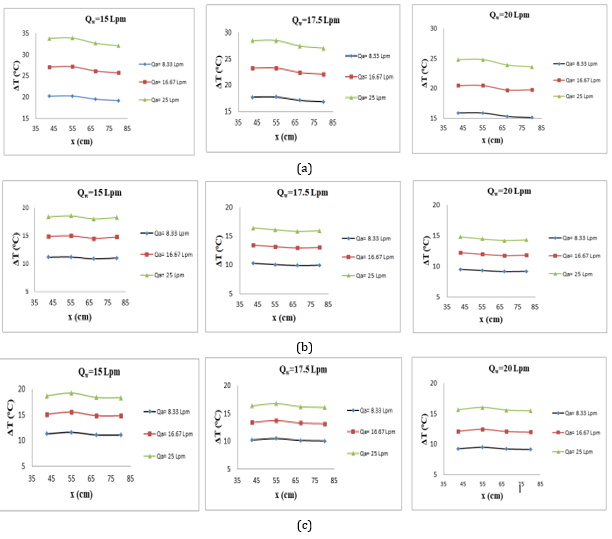Figure 8 The temperature gradient between the flow of water and air with oval tube surfaces for two-phase flow (a) without winglets (b) with Delta winglets (c) with Rectangular winglets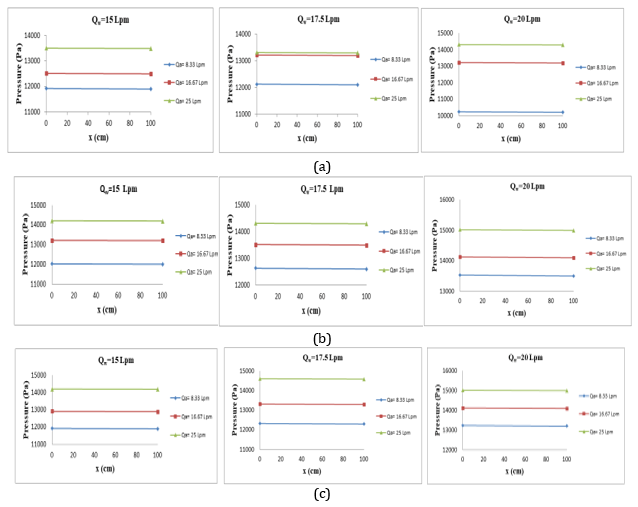Figure 9 Inlet and Outlet pressure in the duct with an oval tube for two-phase flow (a) without winglets (b) with Delta winglets (c) with Rectangular winglets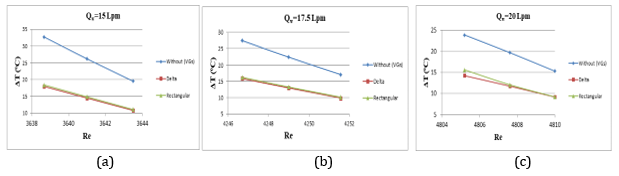Figure 10 The temperature gradient with Delta and Rectangular winglets for two-phase flow over oval tube at (a) Qw= 15 L/min (b) Qw= 17.5 L/min (c) Qw= 20 L/min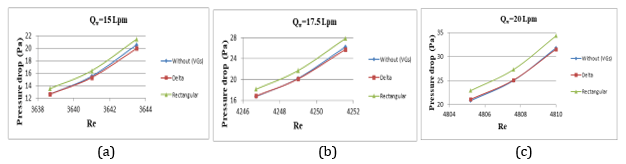Figure 11 Pressure drop with Delta and Rectangular winglets for two-phase flow over oval tube at (a) Qw= 15 L/min (b) Qw= 17.5 L/min (c) Qw= 20 L/min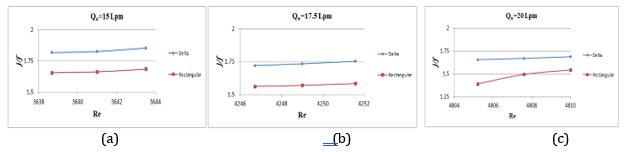Figure 12 Performance parameter with Delta and Rectangular winglets for two-phase flow over oval tube (a) Qw= 15 L/min (b) Qw= 17.5 L/min (c) Qw= 20 L/min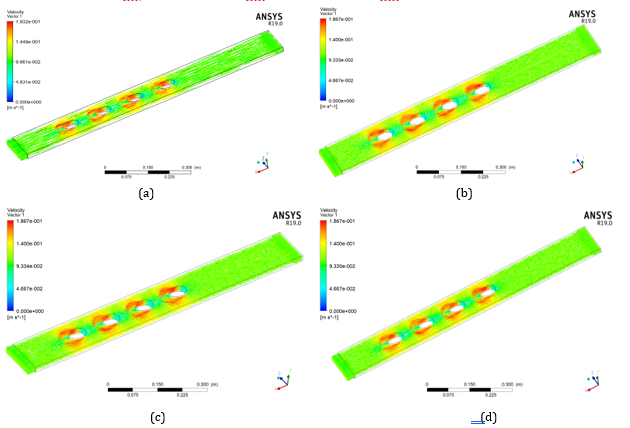Figure 13 The velocity vector without vortex generators (a) single-phase flow (b) two-phase flow at constant a flow rate of water 15 Lpm with a flow rate of air 8.33 Lpm          (c) 16.67 Lpm (d) 25 Lpm